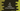# C# program to find the largest of two numbers## C# program to find the largest of two numbers:

In this post, we will learn how to find the largest of two user given numbers. We will take the numbers as inputs from the user and print out a message explaining which one is greater or smaller.

We can solve this problem in two different ways. We can use either an if-else block or ternary operator.

With this post, you will learn how to find the largest of two numbers using if-else and ternary operator in C#.

### Syntax of if-else and ternary operator:

Below is the syntax of if-else condition:

``````if(a > b){

}else{

}``````

where, a and b are the numbers that we are taking for comparison. If a is greater than b, then it will run the code that we will write inside the {} after if block. Else, it will execute the code inside the {} after else block.

And, below is the syntax of ternary operator:

``(a > b) ? "a is greater than b" : "a is smaller than b"``

From the texts, you can understand how ternary opertor works. If a is greater than b, than it will return the part after ? symbol. Else, it gets the part after :.

### Example of if-else:

Below is the complete example that uses if-else to find the greater number:

``````using System;

public class Program
{
public static void Main()
{
int first,second;

Console.WriteLine("Enter the first number : ");

Console.WriteLine("Enter the second number : ");

if(first > second){
Console.WriteLine("First number is greater than the second number");
}else{
Console.WriteLine("First number is smaller than the second number");
}
}
}``````

Here,

• we are storing the first and the second number in first and second integer variables.
• Using a if-else block, we are checking which number is the largest and based on that we are printing one message.

It will print outputs as like below:

``````Enter the first number :
12
Enter the second number :
22
First number is smaller than the second number

Enter the first number :
12
Enter the second number :
11
First number is greater than the second number``````

### Example of ternary operator:

Similarly, we can also use a ternary operator to find the larger number and print one message based on it. Below program explains that:

``````using System;

public class Program
{
public static void Main()
{
int first,second;

Console.WriteLine("Enter the first number : ");